# Abstract Witt ring

(diff) ← Older revision | Latest revision (diff) | Newer revision → (diff)

There are many definitions of an abstract Witt ring. They all seek to define a class of rings that includes Witt rings of fields (of characteristic not two) and that is closed under fibre products, extensions by groups of exponent two and certain quotients. The need for such a class of rings became apparent early in the (still incomplete) classification of Noetherian Witt rings of fields.

Two series of definitions, that of J. Kleinstein and A. Rosenberg [a1] and M. Marshall [a2], led to the same class of rings, which is now the most widely used. In this sense, an abstract Witt ring is a pairwhere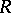is a commutative ring with unitandis a subgroup of the multiplicative groupwhich has exponent two, containsand generatesadditively. Let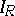denote the ideal ofgenerated by elements of the form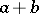, with. It is further assumed that:

1) if, then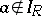;

2) ifand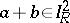, then;

3) if, withand all, then there exist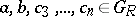such thatand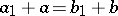.

Whenis the Witt ring of a field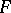, then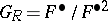and property 3) is a consequence of the Witt cancellation theorem.

How to Cite This Entry:
Abstract Witt ring. Encyclopedia of Mathematics. URL: http://encyclopediaofmath.org/index.php?title=Abstract_Witt_ring&oldid=16610
This article was adapted from an original article by R.W. Fitzgerald (originator), which appeared in Encyclopedia of Mathematics - ISBN 1402006098. See original article